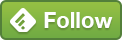## Sep 30 How To Calculate Month-On-Month Change In Website Traffic In R Studio

In order to plot this chart, what you’ll need:

ggplot

dplyr package + mutate function to create a calculated field [% change in monthly sessions]

In order to get the end result like this:

We first use the GoogleAnalyticsR package to pull the data from GA. Here, I have Sessions + yearMonth data for all of 2018.

We then call the library dplyr and use the mutate function to create a calculated field [month-on-month change] that shows the % values.

```library(dplyr)
#new column added using a calculated field
mutate(mom_per_change = (sessions - lag(sessions))/lag(sessions))
```

As you can see in the chart, the y axis has the change in decimal format instead of percentages.

Adding the scale_y_continuous parameter to the ggplot now converts the y axis to percentage format.

```#number format changed to show Y axis as %
y=mom_per_change)) +
geom_col() +
scale_y_continuous(labels = scales::percent)```

```#Full code
library("ggplot2")

ga_auth()
ga_account_list()

ga_id <- 1234567

date_range = c("2018-01-01","2018-09-21"),
metrics = c("sessions"),
dimensions = c("yearMonth"),
anti_sample = TRUE)

library(dplyr)

#new column added using a calculated field
mutate(mom_per_change =
(sessions - lag(sessions))/lag(sessions))

#Plot created to show % change
y=mom_per_change)) +
geom_col()

#number format changed to show Y axis as %